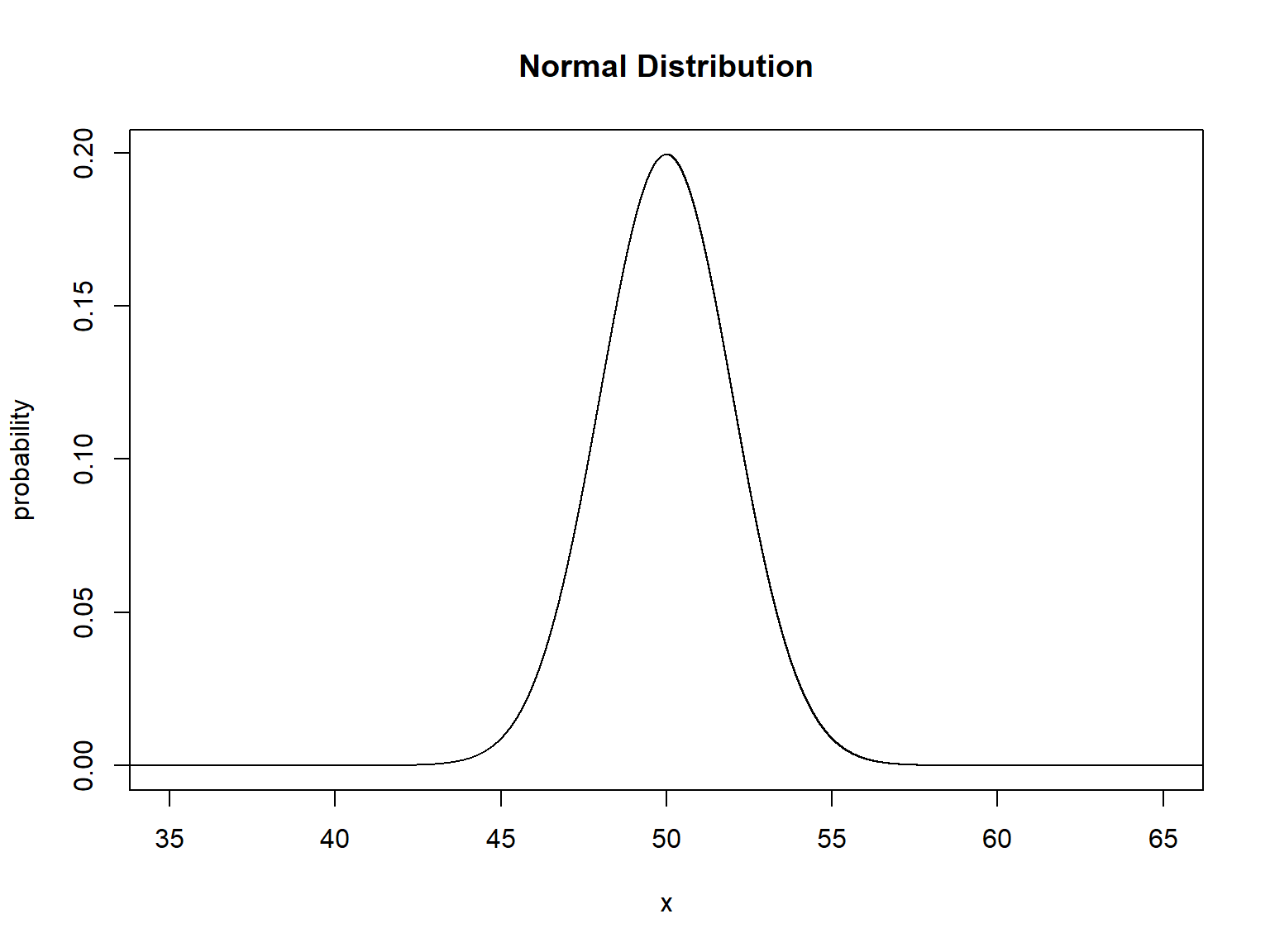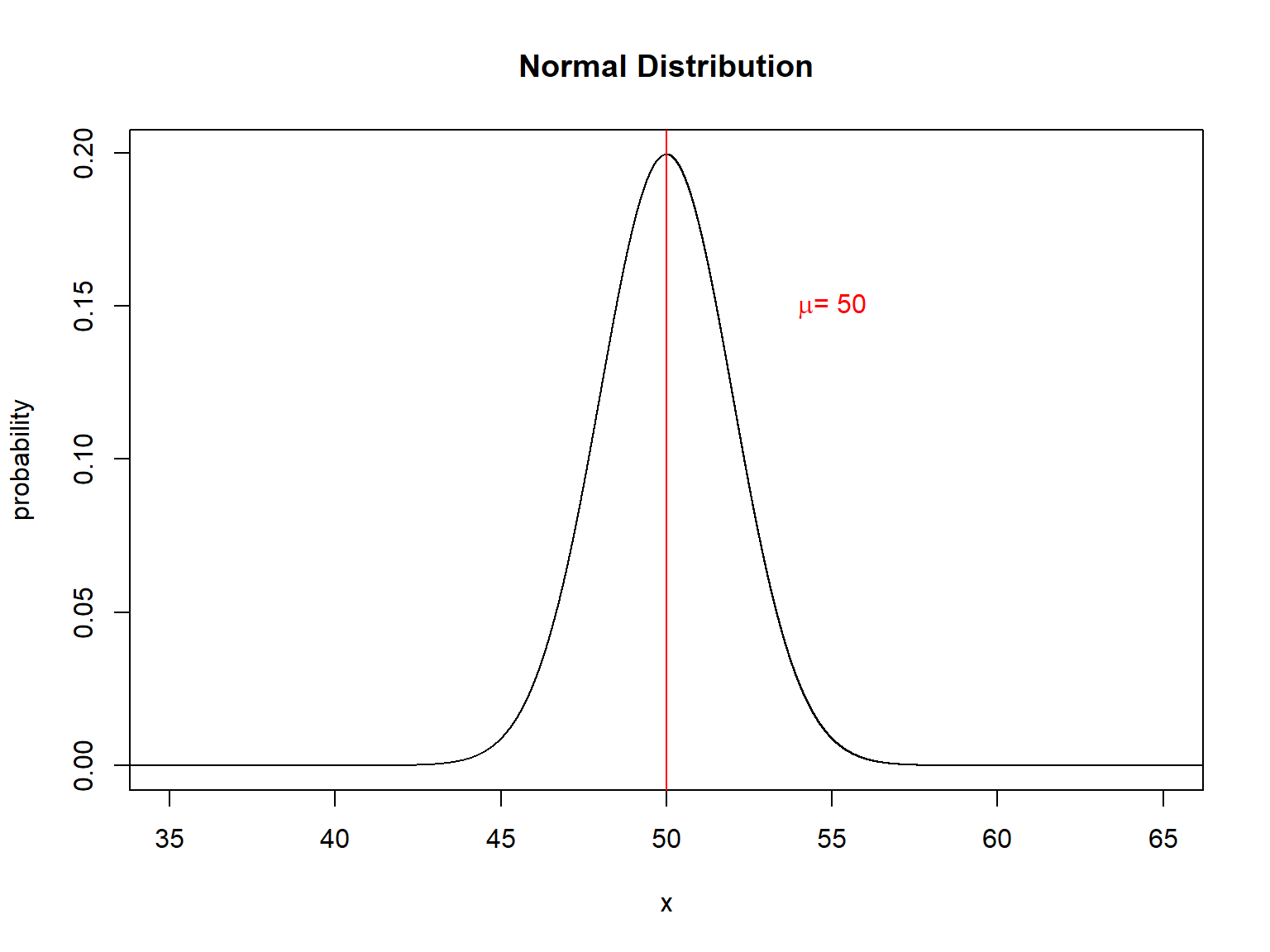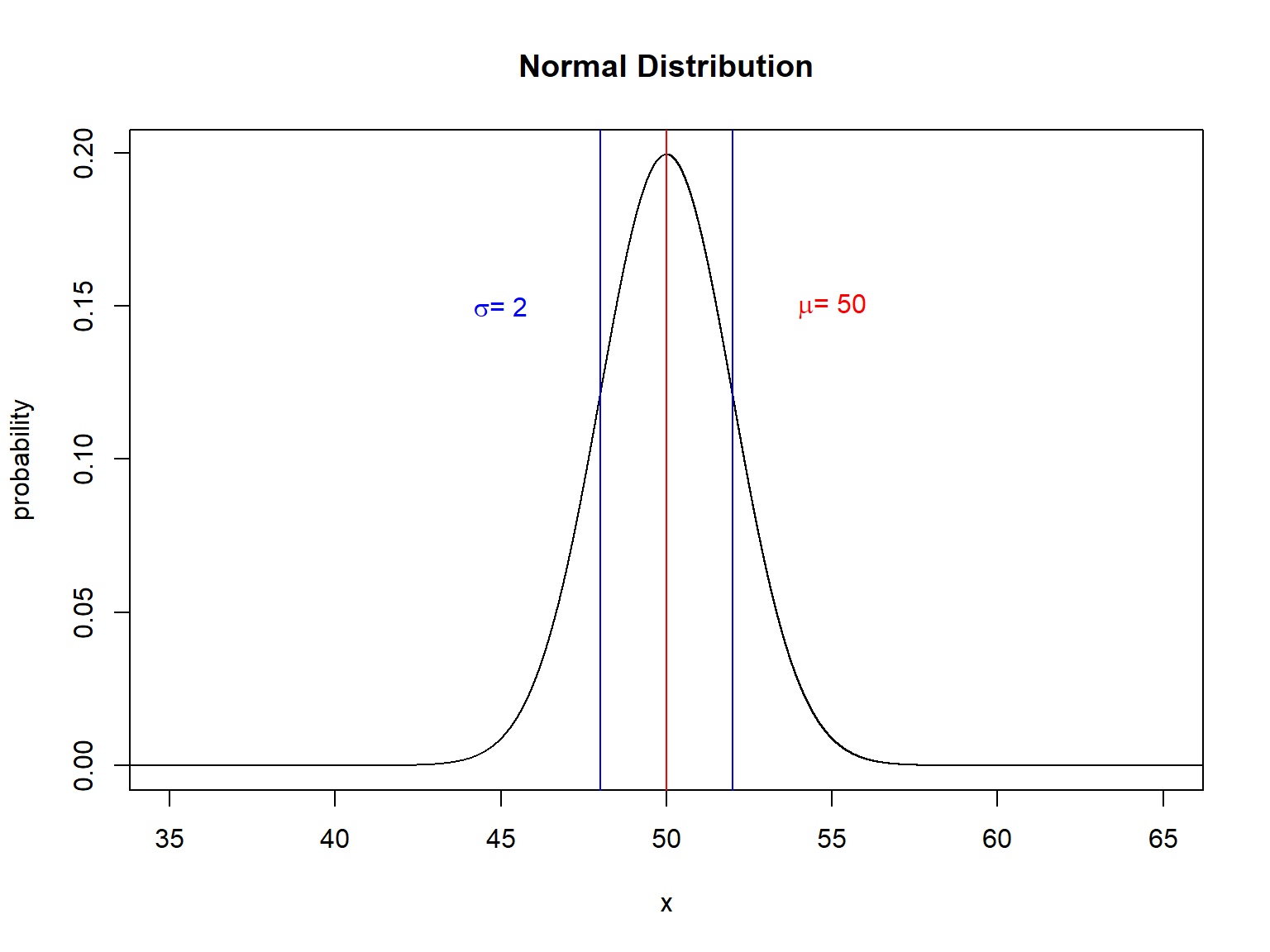March 26, 2018

# Learning Objectives

• Be able to describe the charateristics of the normal distribution
• Be able to use the mean and standard deviation to describe any normal distribution
• Be able to convert a normally distributed variable to the “standard normal distribution” and calculate a Z score.
• Get p values from a standard anormal table (or equivalent procedure in R).
• Relate the Central Limit Theorem (CLT) to sampling distributions
• Relate the normal and binomial distributions

# The Normal Distribution# The Normal Distribution# The Normal Distribution# Normal Distribution Characteristics

• Continuous variables
• Positive or negative numbers $$-\infty$$ to $$\infty$$
• Symetrical
• Described using $$\mu$$ and $$\sigma$$
• The normal probability density function: $\frac{1}{\sqrt{2 \pi \sigma^{2}}} e^{- \frac{(x- \mu )^{2}}{2 \sigma ^{2}}}$
You don’t need to memorize this, but note how this is functionally equivalent to the binomial function

# An example (with code!)

Let’s work an example with owl masses (from my research).